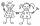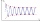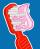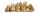# Equation - 6th grade (11y) - math problems - page 12

1. Simple equation 5Solve equation with fractions: X × 3/8 = 1/2
2. ClothesDanka and Janka collect clothes. Danka had 9 more than Janka, so she gave her 7. Which now has more cloth and how many?
3. Seven timesWhich number seven times is just as higher as 27, how much is smaller than 29?
4. Dropped sheetsThree consecutive sheets dropped from the book. The sum of the numbers on the pages of the dropped sheets is 273. What number has the last page of the dropped sheets?
5. Number with onesThe first digit of the number is 1, if we move this digit to the end we get a 3 times higher number, which is the number?
6. Equation 29Solve next equation: 2 ( 2x + 3 ) = 8 ( 1 - x) -5 ( x -2 )
7. Equation 15Solve equation with variables on both sides:
8. Two thirdsFind two-thirds of the number equal to two-thirds of 99
9. Negative in equation2x + 3 + 7x = – 24, what is the value of x?
10. Brick weightHalf the weight of a brick plus 20 pounds is equal to 1/3 the weight of the brick plus 30 pounds. How much does the brick weigh?
11. Sum of four numbersThe sum of four consecutive natural numbers is 114. Find them.
12. Bed timeTiffany was 5 years old; her week night bedtime grew by ¼ hour each year. If, at age 18, her curfew time is 11pm, what was her bed time when she was 5 years old?
13. Chewing gumsFor 3 chewing gums, you will pay 20 CZK less than 7 chewing gums. How much is 1 chewing gum and how much does a 5pcs package cost?
14. Unknown numberI think the number. I'll reduce it to its one-third. The result is then increased by one-third, and I get the number 12.
15. A manA man spends 5/9 of his money on rent, and 5/16 of the remainder on electricity. If the final balance remaining is 550 find how much was spent on rent
16. Last pageTwo consecutive sheets dropped out of the book. The sum of the numbers on the sides of the dropped sheets is 154. What is the number of the last page of the dropped sheets?
17. TwentyTwenty rabbits are put in 4 cells so that there are different number of rabbits in each cell contains at least 3 rabbits. What is the largest possible number of rabbits in one cell
18. EquatiomSolve equation with negatives: X/(-5) + 2 = -9
19. Brother and sisterWhen I was 8, my sister was 4, now I am 18, how old is my sister?
20. Find xSolve: if 2(x-1)=14, then x= (solve an equation with one unknown)

Do you have an interesting mathematical word problem that you can't solve it? Enter it, and we can try to solve it.

To this e-mail address, we will reply solution; solved examples are also published here. Please enter the e-mail correctly and check whether you don't have a full mailbox.

Please do not submit problems from current active competitions such as Mathematical Olympiad, correspondence seminars etc...

Do you have a linear equation or system of equations and looking for its solution? Or do you have quadratic equation?